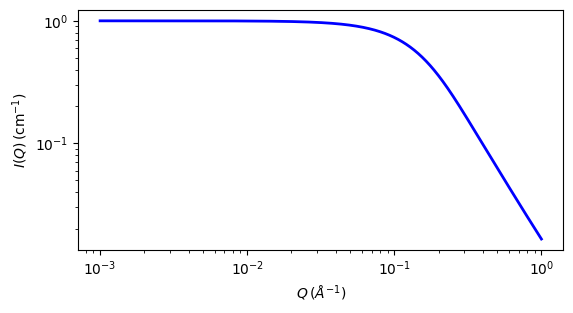# star_polymer

Star polymer model with Gaussian statistics

Parameter

Description

Units

Default value

scale

Scale factor or Volume fraction

None

1

background

Source background

cm-1

0.001

rg_squared

Ensemble radius of gyration SQUARED of the full polymer

2

100

arms

Number of arms in the model

None

3

The returned value is scaled to units of cm-1 sr-1, absolute scale.

Definition

Calcuates the scattering from a simple star polymer with f equal Gaussian coil arms. A star being defined as a branched polymer with all the branches emanating from a common central (in the case of this model) point. It is derived as a special case of on the Benoit model for general branched polymers as also used by Richter et al.

For a star with $$f$$ arms the scattering intensity $$I(q)$$ is calculated as

$I(q) = \frac{2}{fv^2}\left[ v-1+\exp(-v)+\frac{f-1}{2} \left[ 1-\exp(-v)\right]^2\right]$

where

$v=\frac{uf}{(3f-2)}$

and

$u = \left\langle R_{g}^2\right\rangle q^2$

contains the square of the ensemble average radius-of-gyration of the full polymer while v contains the radius of gyration of a single arm $$R_{arm}$$. The two are related as:

$R_{arm}^2 = \frac{f}{3f-2} R_{g}^2$

Note that when there is only one arm, $$f = 1$$, the Debye Gaussian coil equation is recovered.

Note

Star polymers in solutions tend to have strong interparticle and osmotic effects. Thus the Benoit equation may not work well for many real cases. A newer model for star polymer incorporating excluded volume has been developed by Li et al in arXiv:1404.6269 [physics.chem-ph]. Also, at small $$q$$ the scattering, i.e. the Guinier term, is not sensitive to the number of arms, and hence ‘scale’ here is simply $$I(q=0)$$ as described for the mono_gauss_coil model, using volume fraction $$\phi$$ and volume V for the whole star polymer.Fig. 120 1D plot corresponding to the default parameters of the model.

Source

star_polymer.py $$\ \star\$$ star_polymer.c

References

Authorship and Verification

• Author: Kieran Campbell Date: July 24, 2012